# Rajasthan Board Question Paper for Class 10 Maths 2018 In PDF

## RBSE 10th Maths Question Papers 2018 With Solutions

Rajasthan Board 10th question paper 2018 Maths with solutions are provided on this page in text and downloadable pdf format for the ease of the students. Students can download the official RBSE maths question paper 2018 along with solutions of Class 10 for reference. All the past year papers can be obtained by visiting Rajasthan board previous year maths question papers. These can be downloaded easily and students can practice and verify the answers provided by BYJU’S. Solving RBSE 2018 Maths question papers for class 10th familiarizes the students with the pattern of the question paper and the type of questions that will appear in the board examination.

### QUESTION PAPER CODE S–09–Mathematics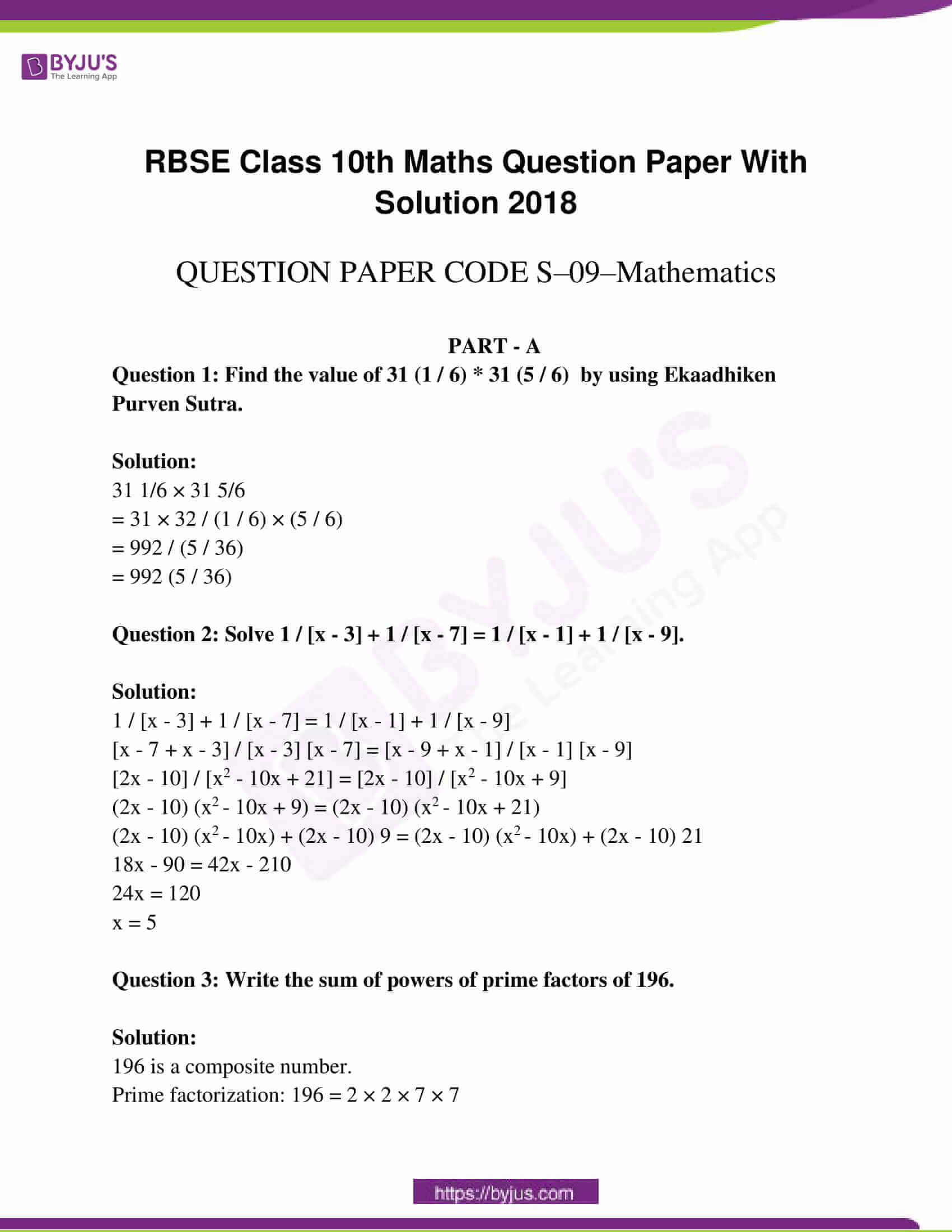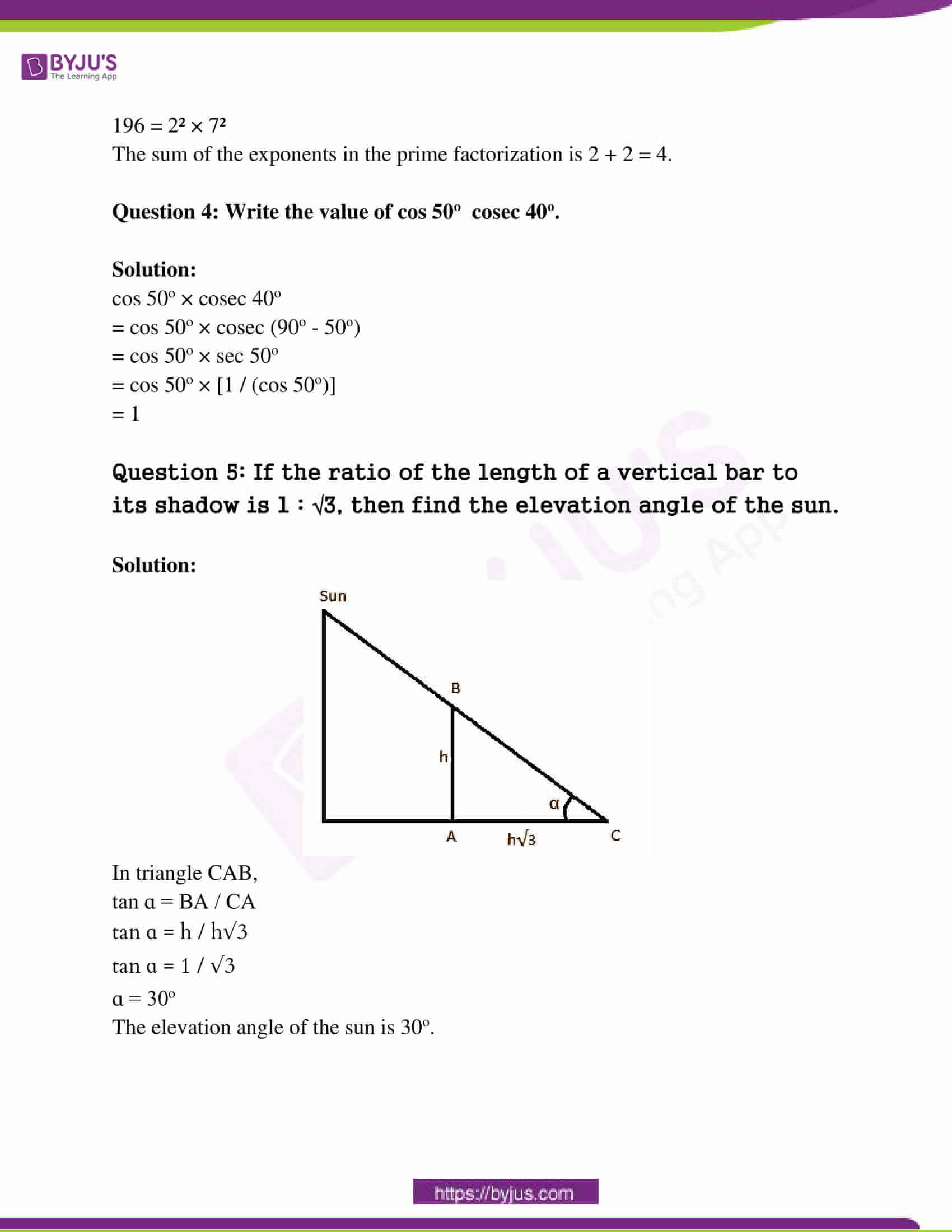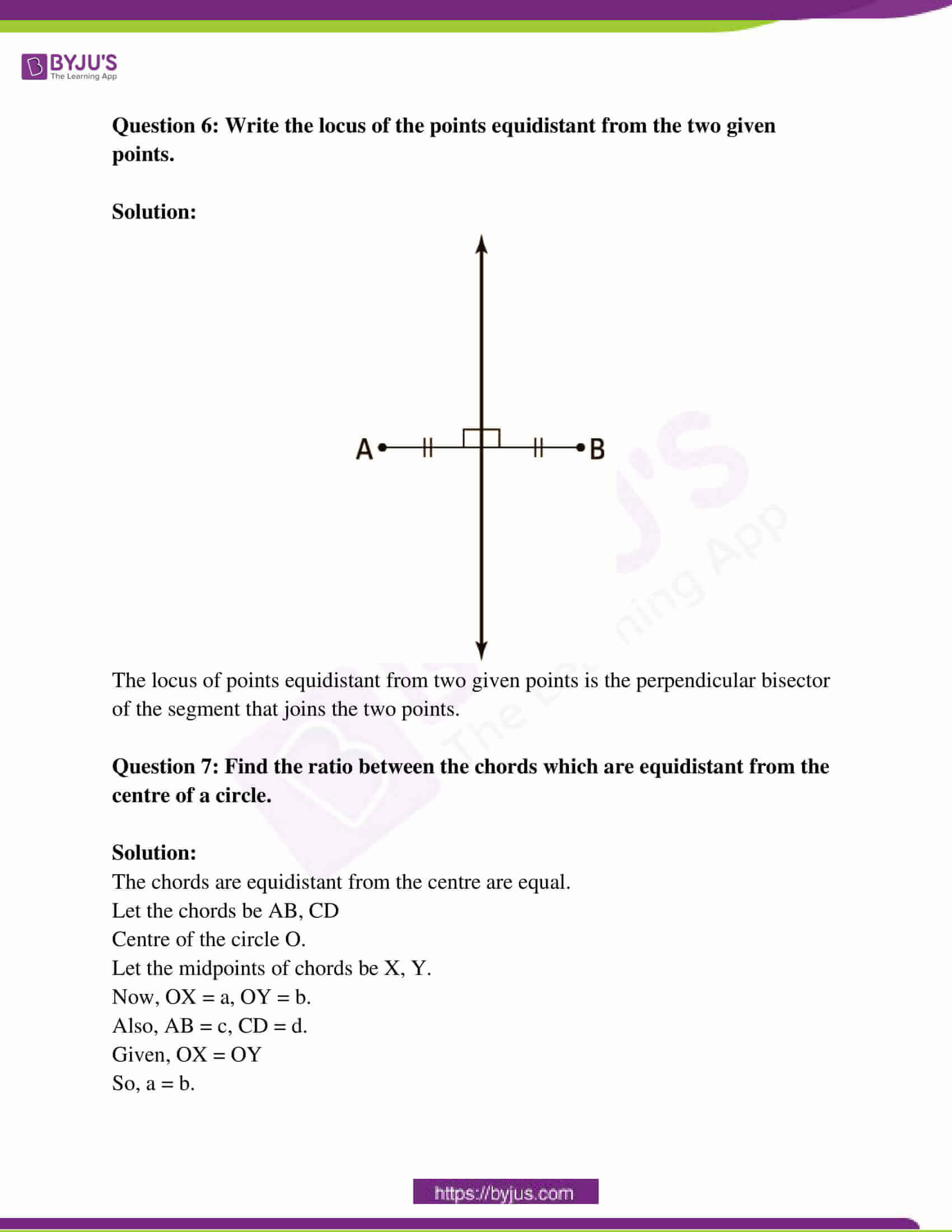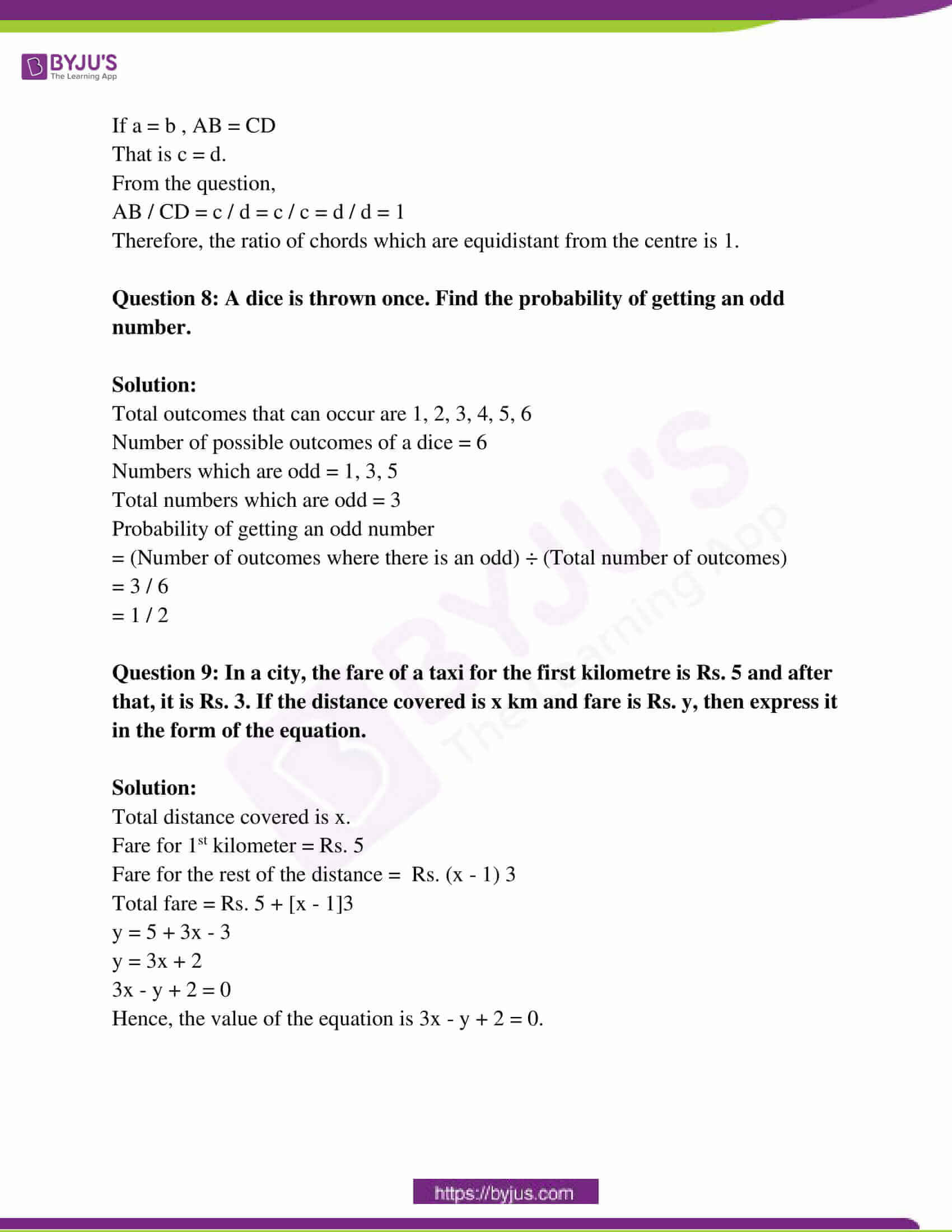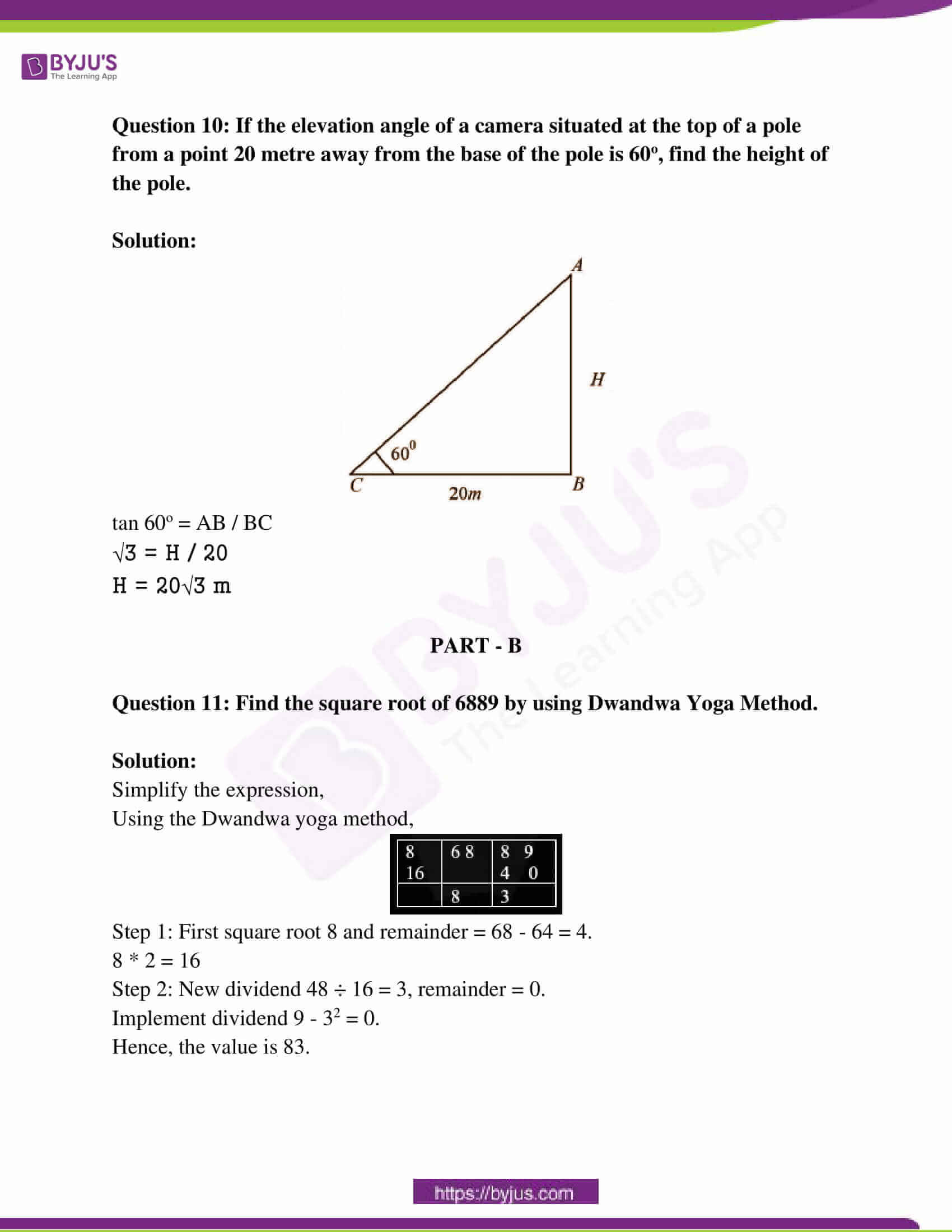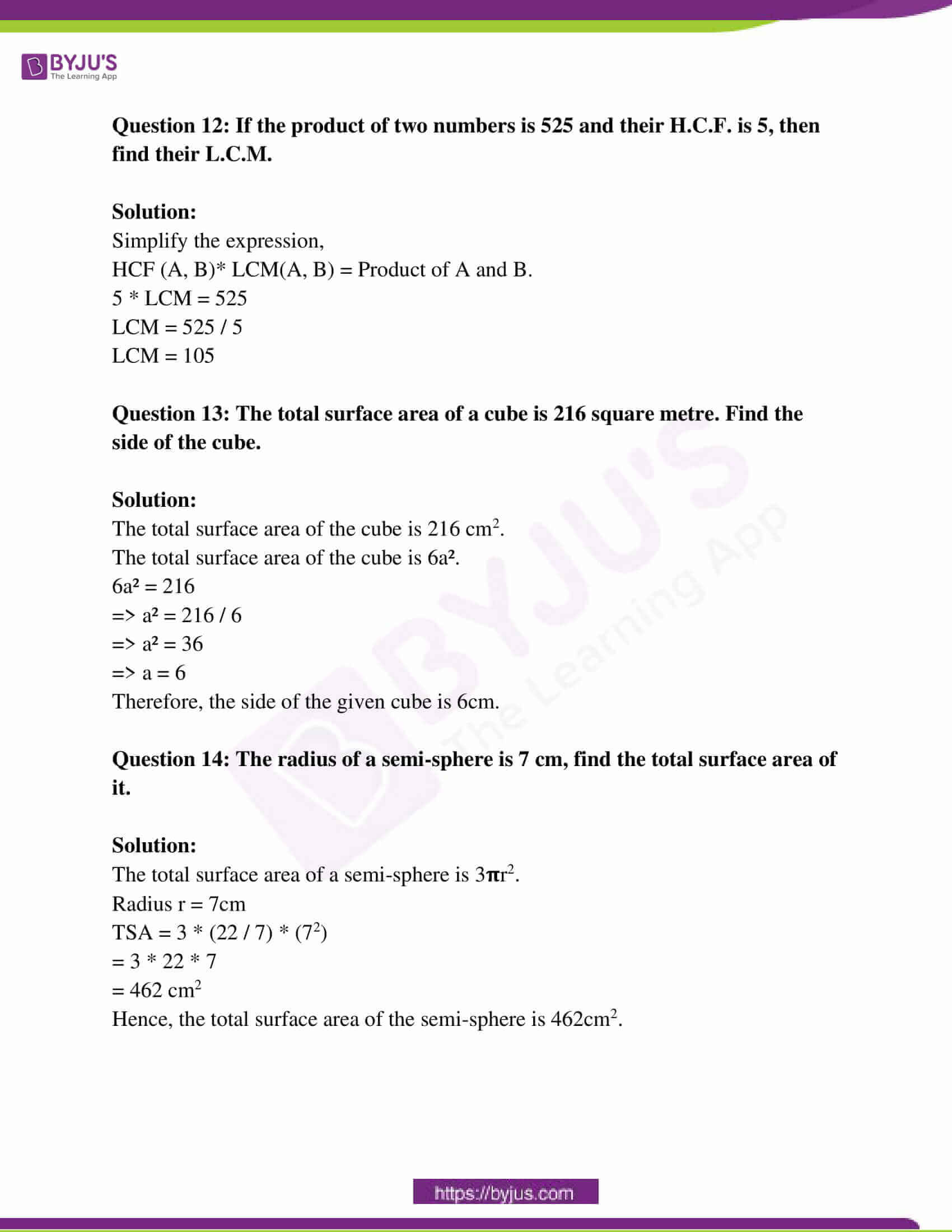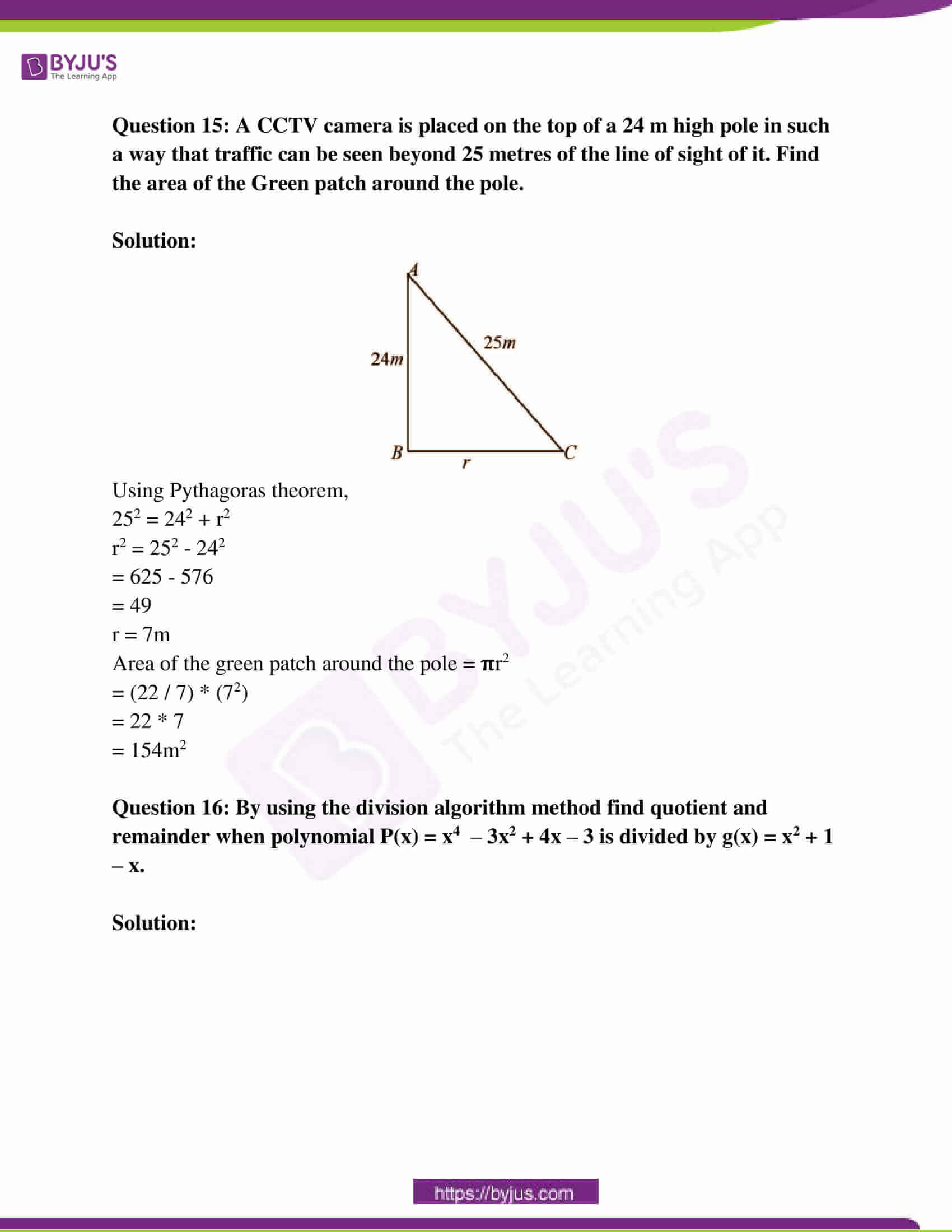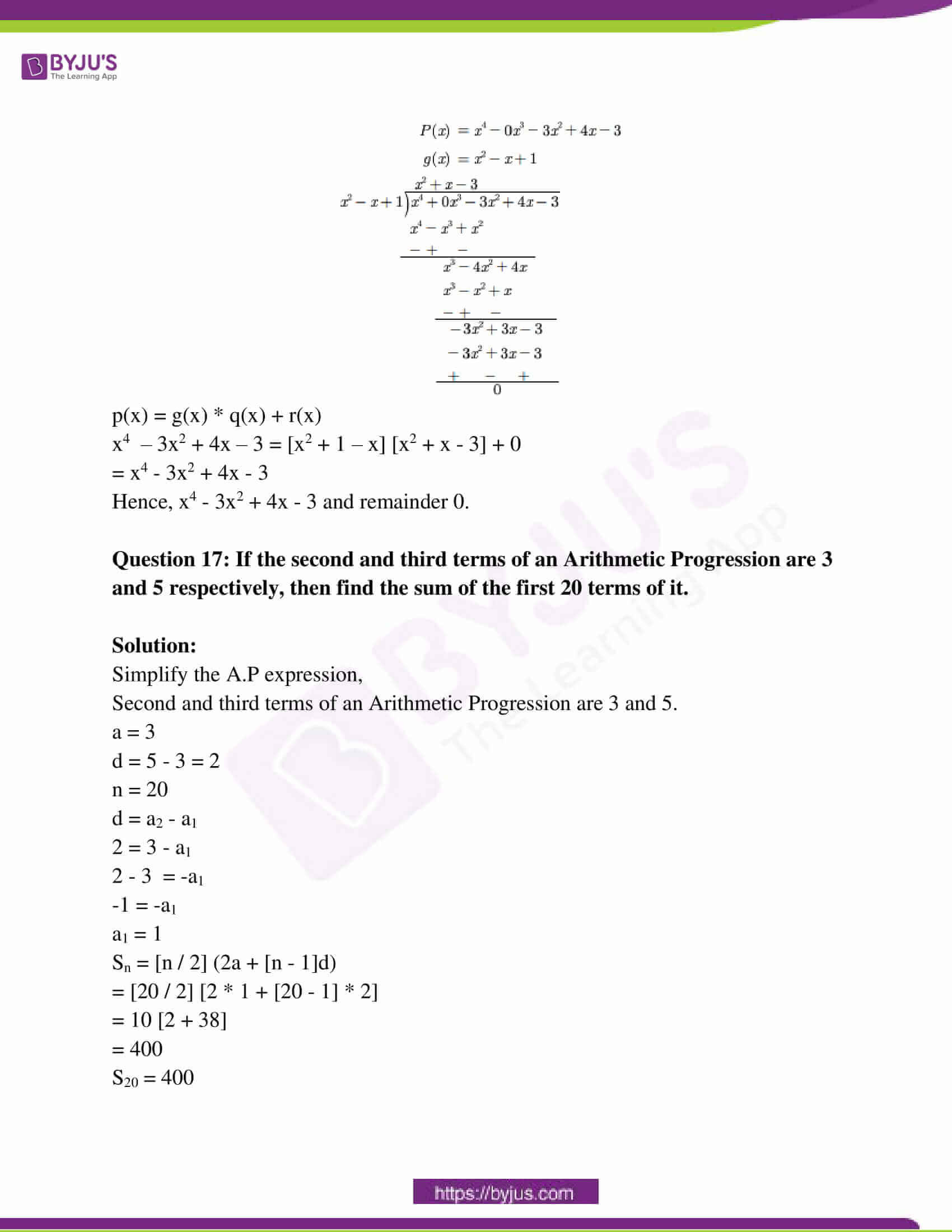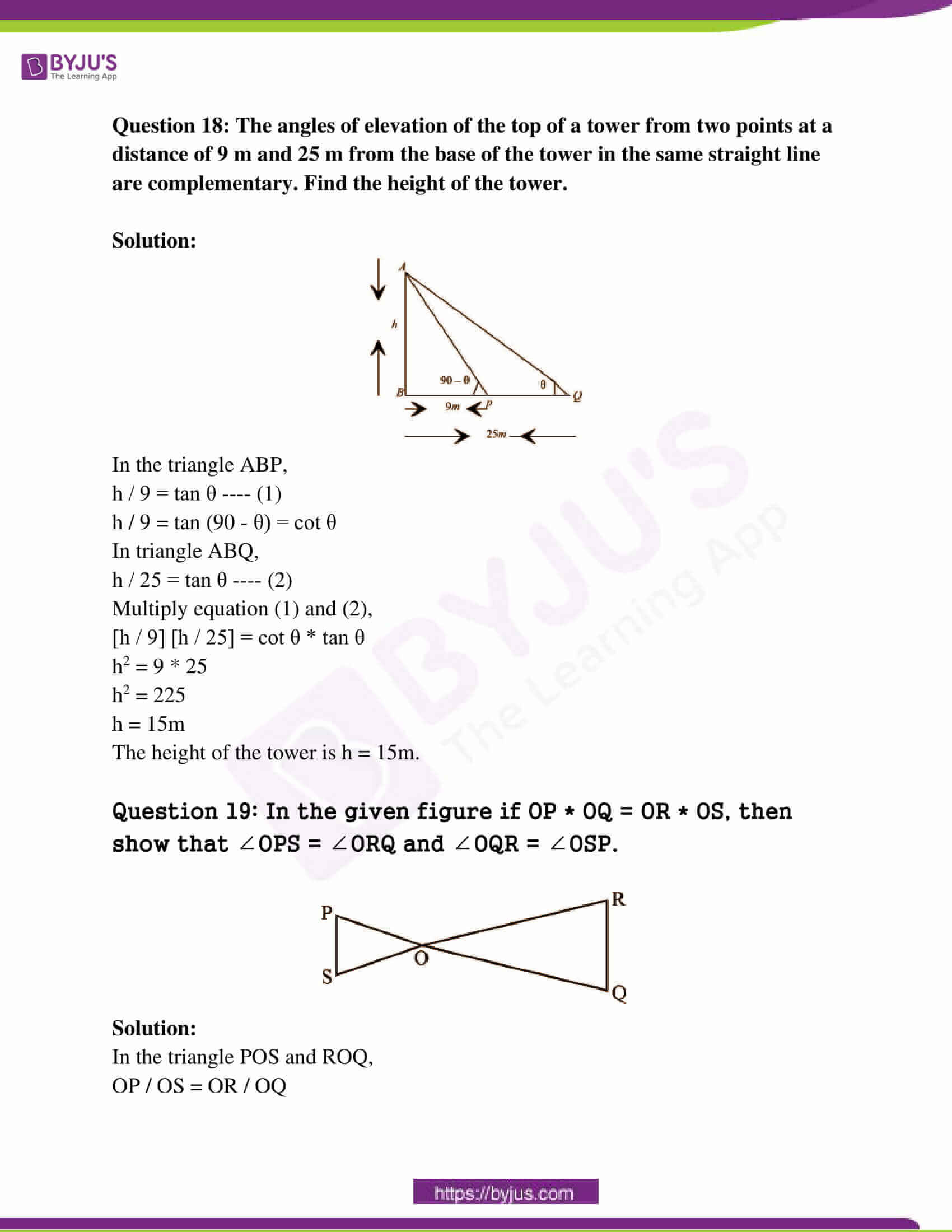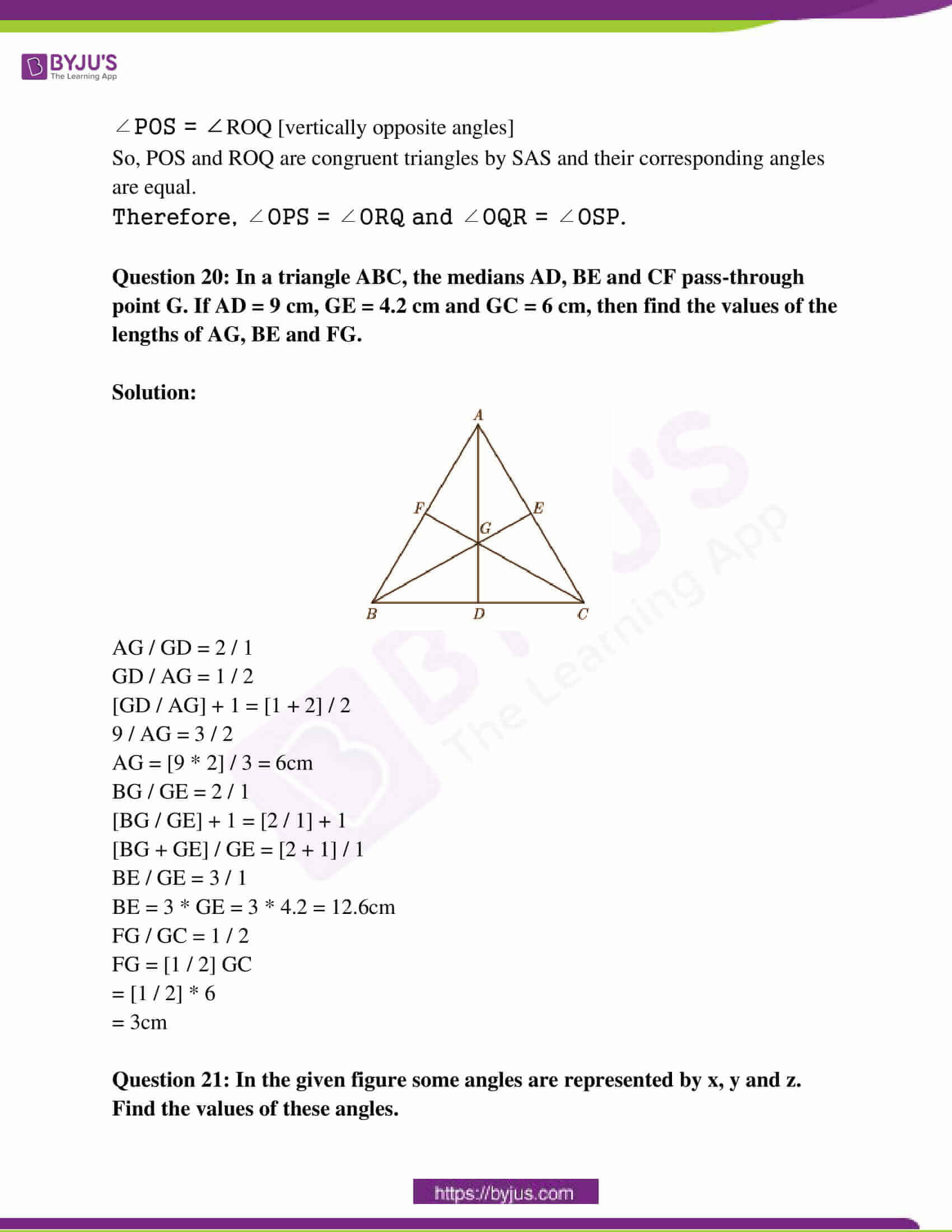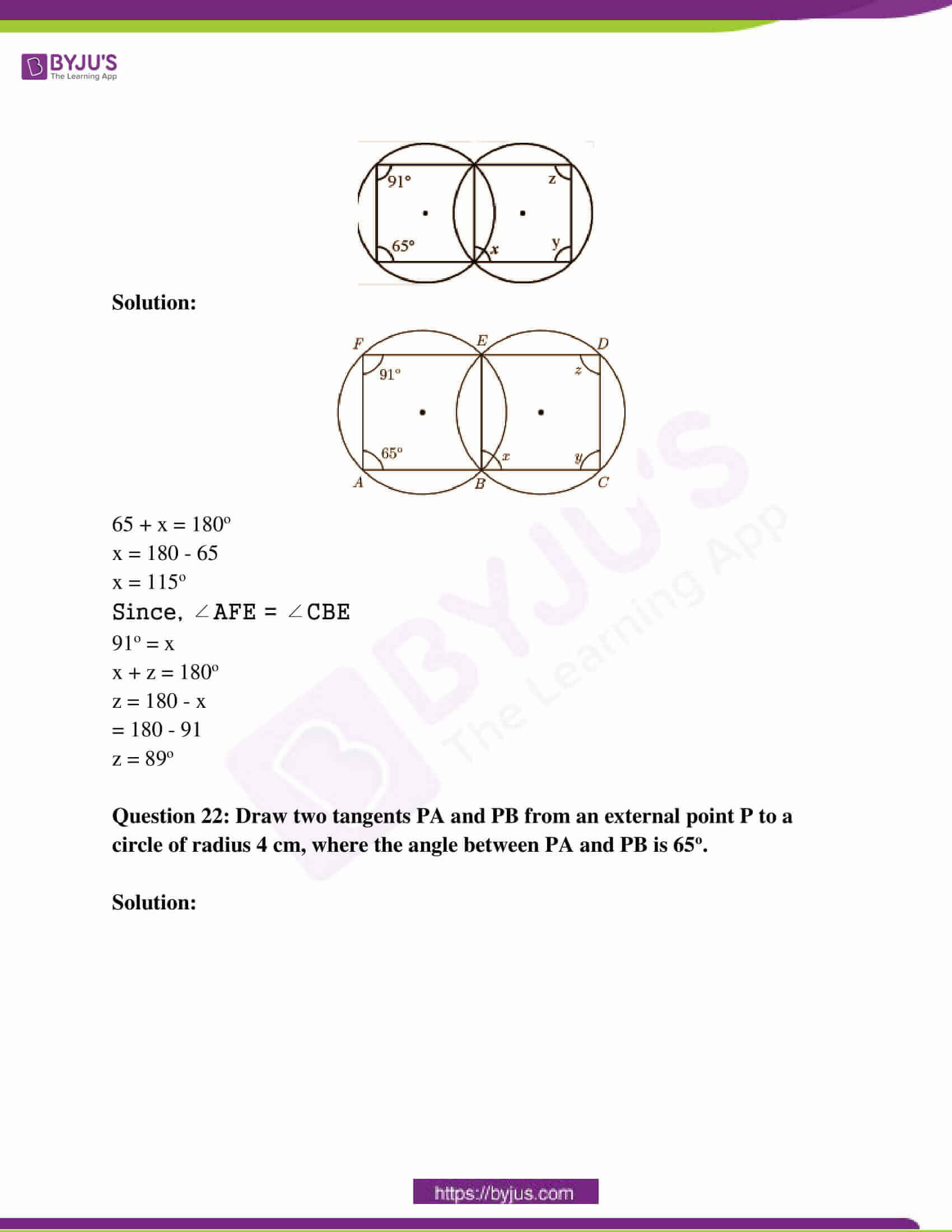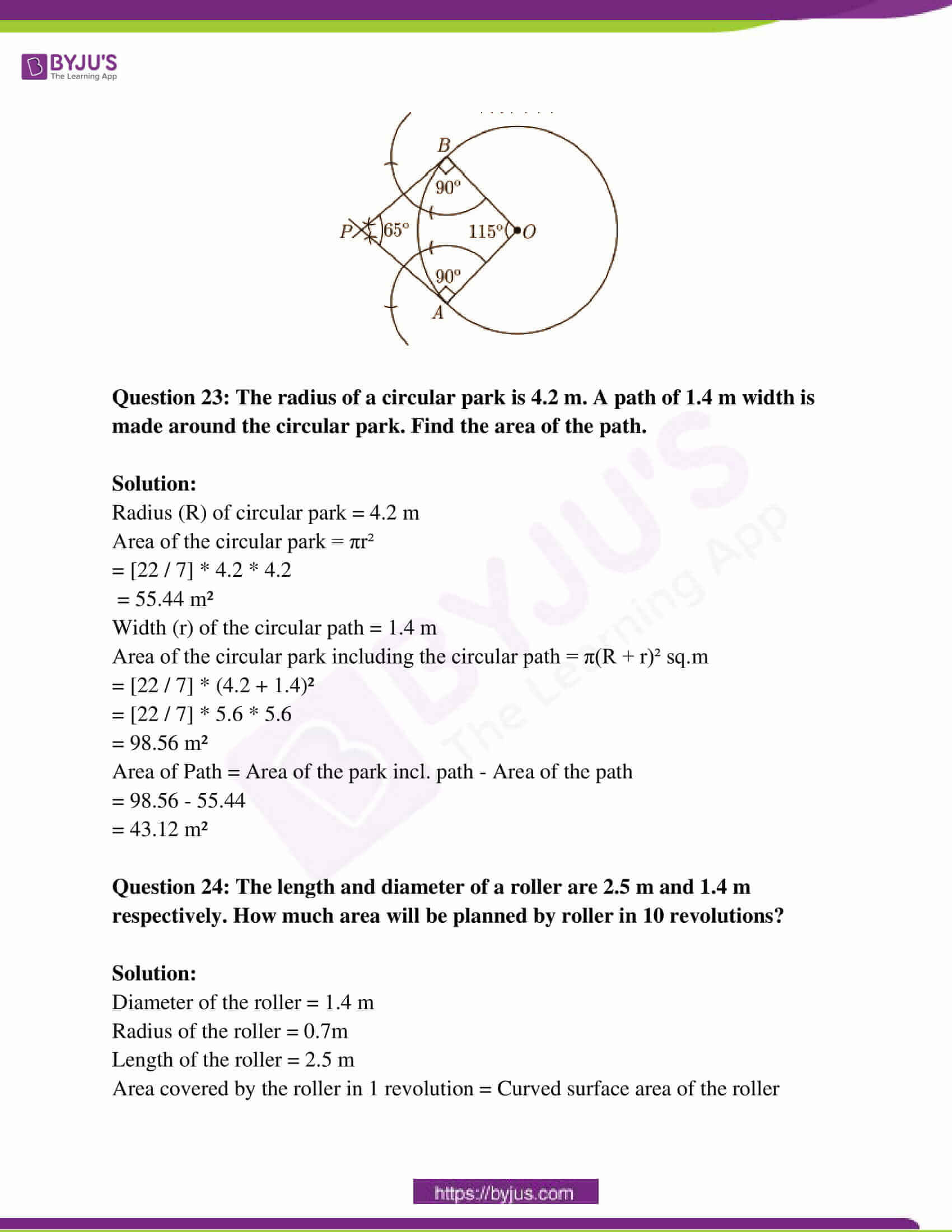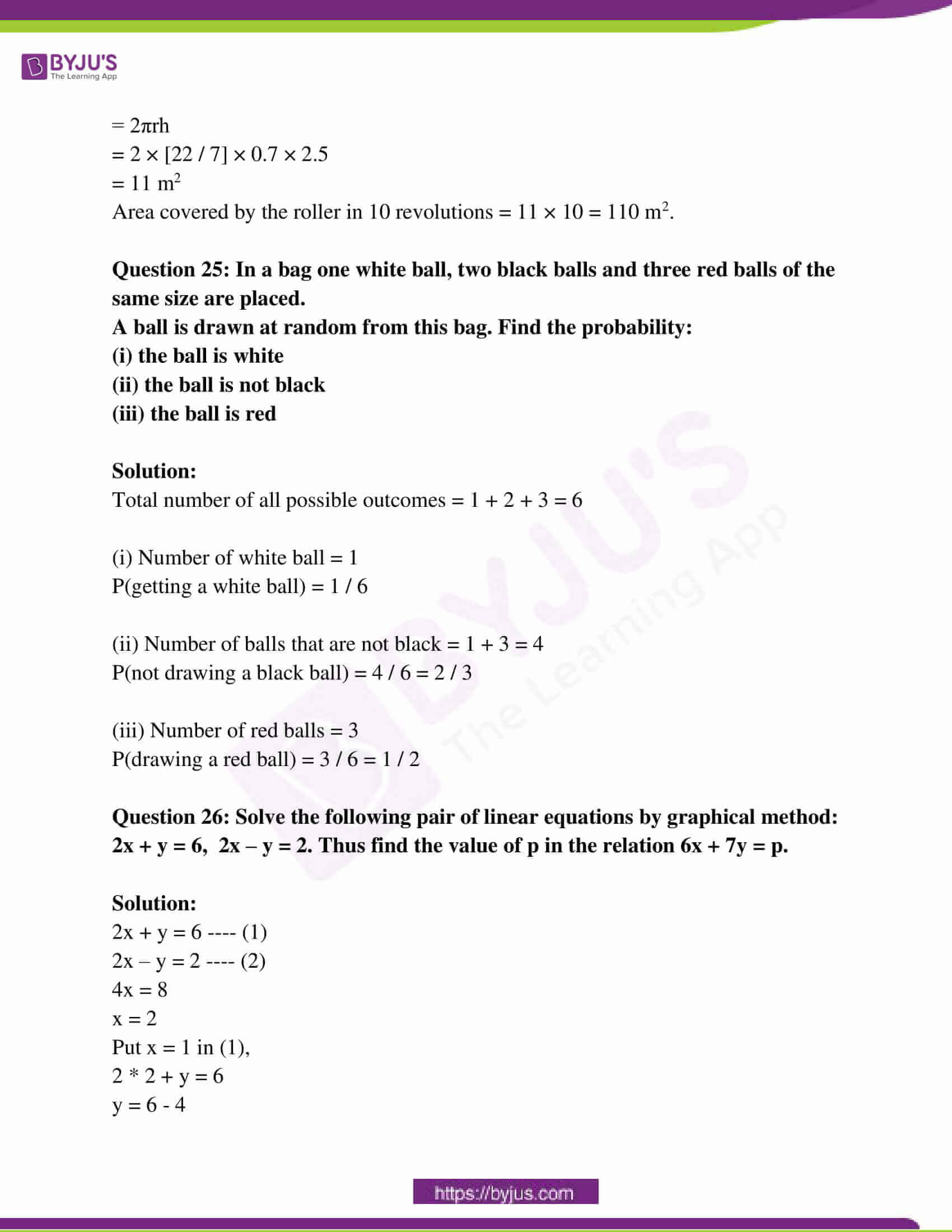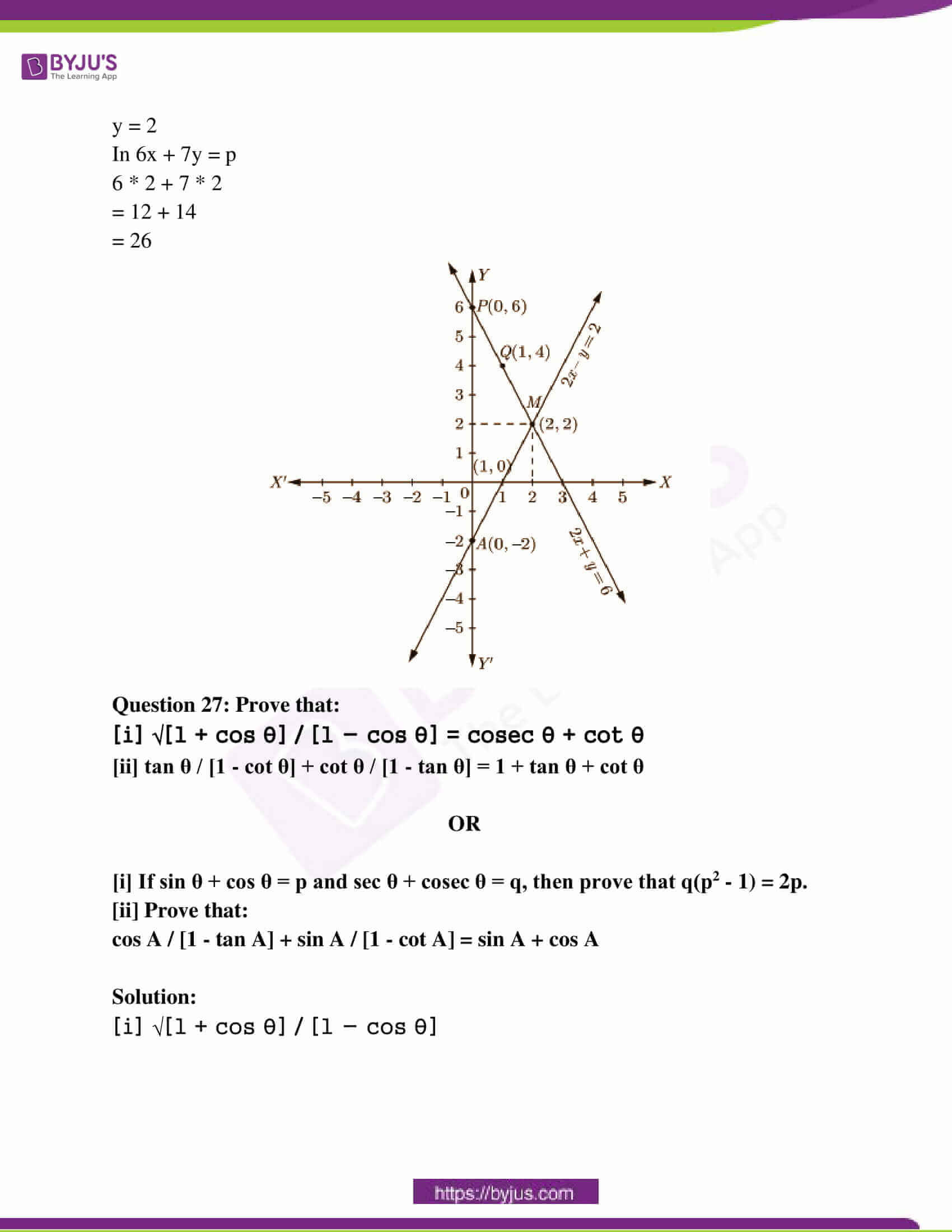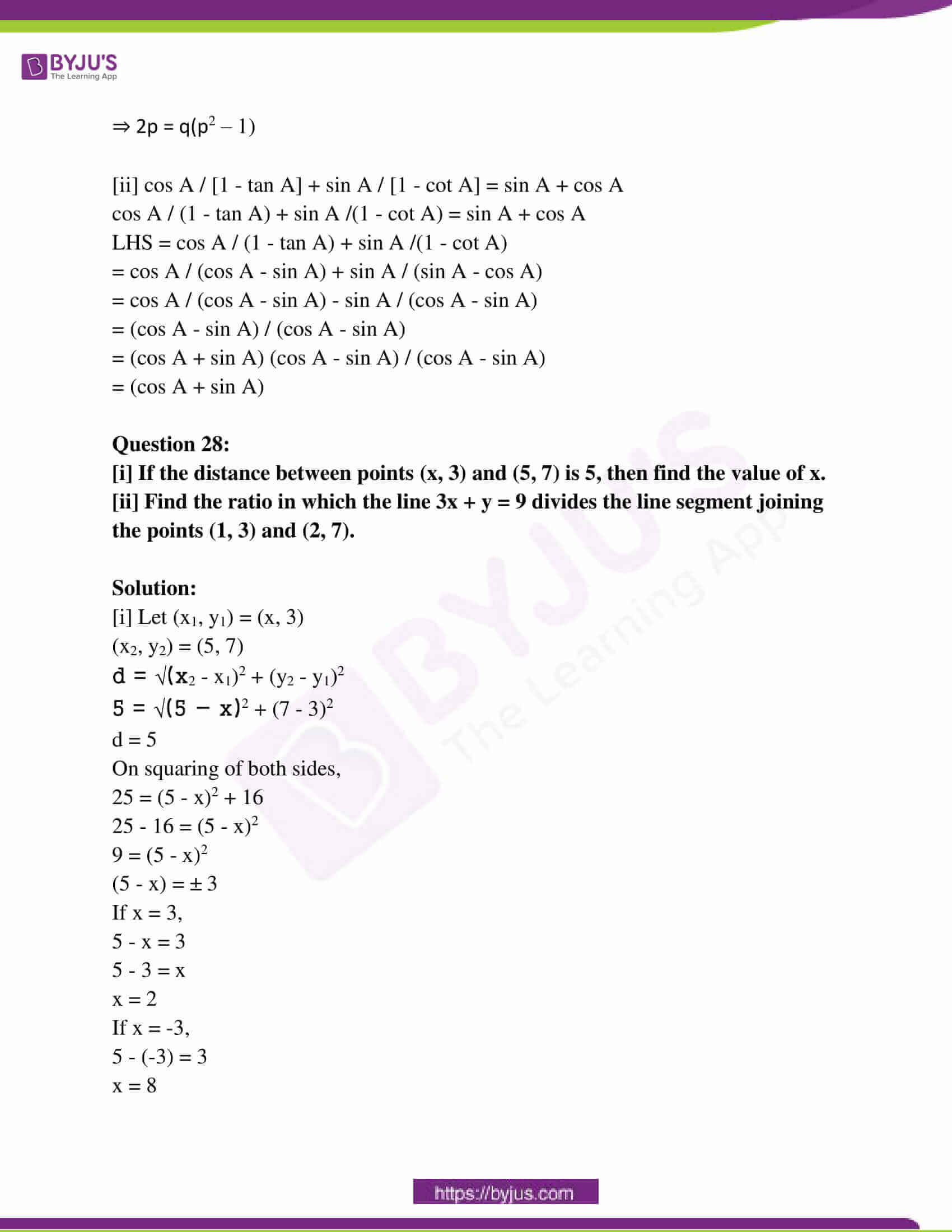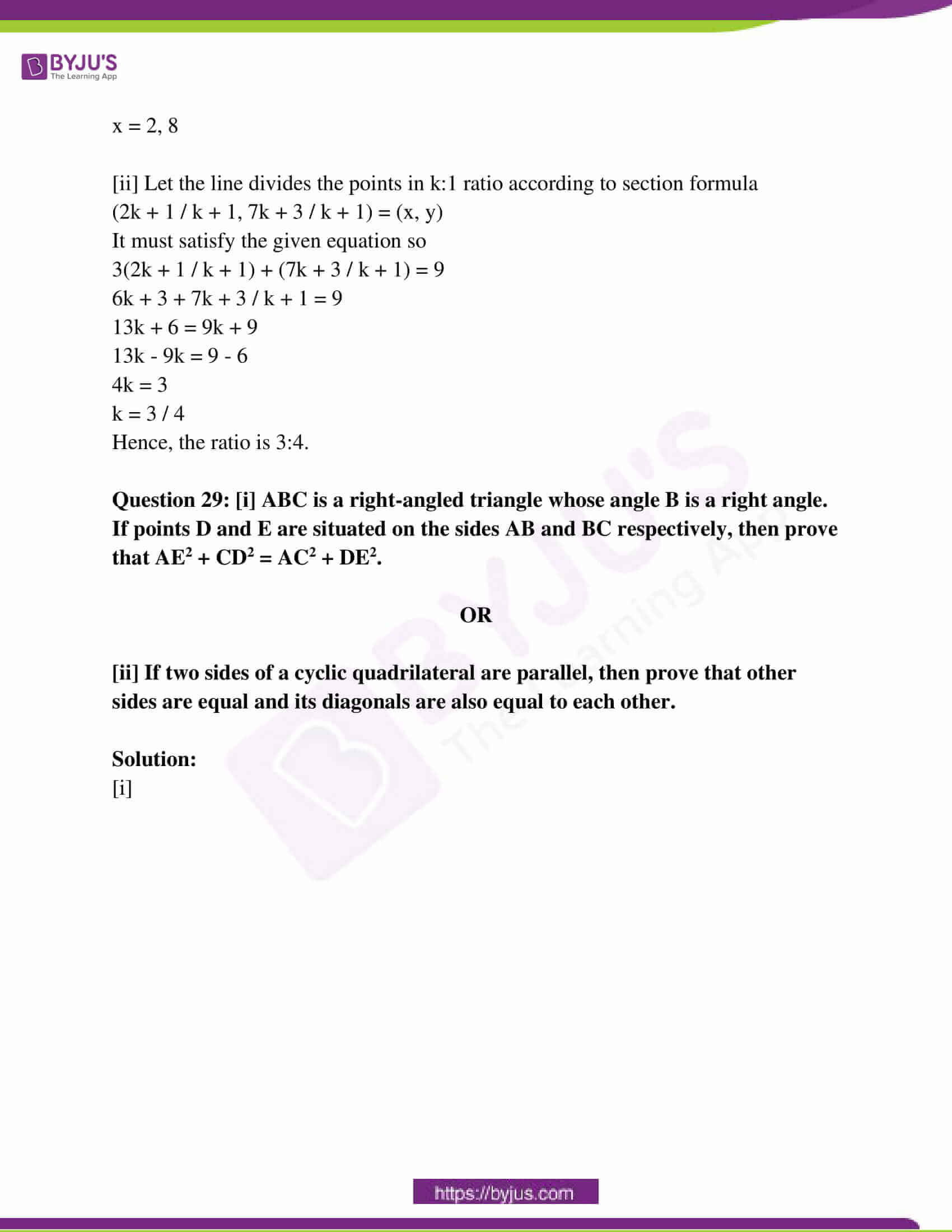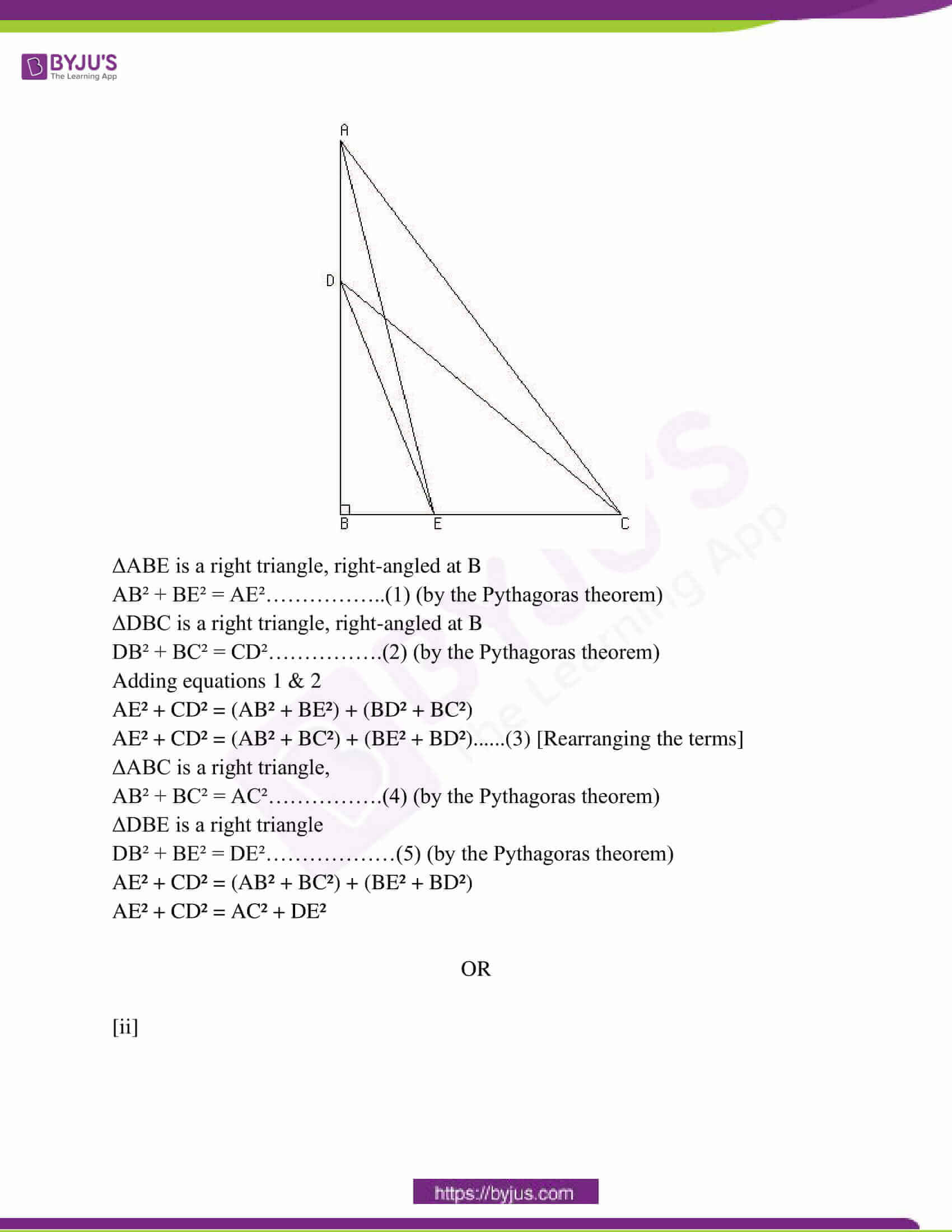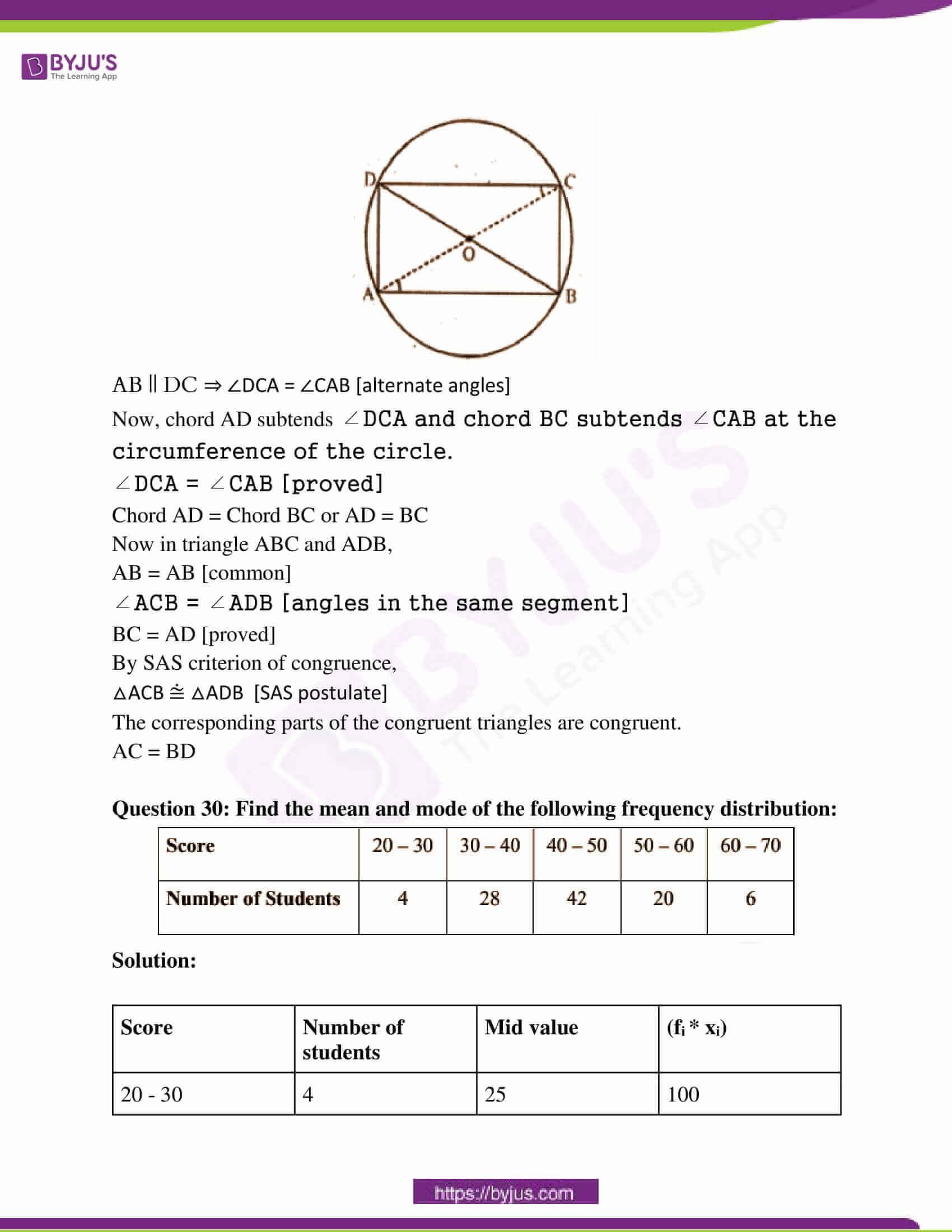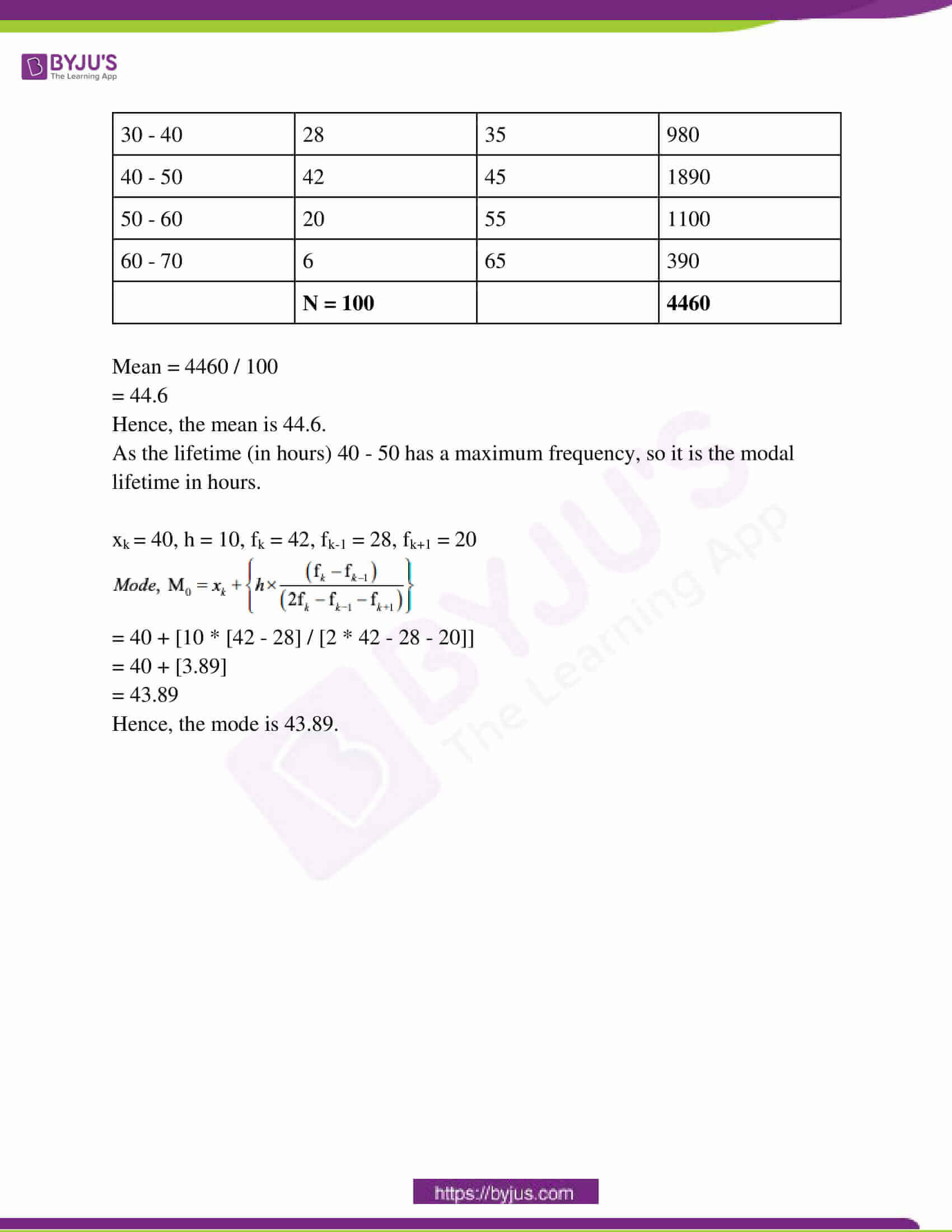### RBSE Class 10th Maths Question Paper With Solutions 2018

PART – A

Question 1: Find the value of 31 (1 / 6) * 31 (5 / 6) by using Ekaadhiken Purven Sutra.

Solution:

31 1/6 × 31 5/6

= 31 × 32 / (1 / 6) × (5 / 6)

= 992 / (5 / 36)

= 992 (5 / 36)

Question 2: Solve 1 / [x – 3] + 1 / [x – 7] = 1 / [x – 1] + 1 / [x – 9].

Solution:

1 / [x – 3] + 1 / [x – 7] = 1 / [x – 1] + 1 / [x – 9] [x – 7 + x – 3] / [x – 3] [x – 7] = [x – 9 + x – 1] / [x – 1] [x – 9] [2x – 10] / [x2 – 10x + 21] = [2x – 10] / [x2 – 10x + 9]

(2x – 10) (x2 – 10x + 9) = (2x – 10) (x2 – 10x + 21)

(2x – 10) (x2 – 10x) + (2x – 10) 9 = (2x – 10) (x2 – 10x) + (2x – 10) 21

18x – 90 = 42x – 210

24x = 120

x = 5

Question 3: Write the sum of powers of prime factors of 196.

Solution:

196 is a composite number.

Prime factorization: 196 = 2 × 2 × 7 × 7

196 = 2² × 7²

The sum of the exponents in the prime factorization is 2 + 2 = 4.

Question 4: Write the value of cos 50o cosec 40o.

Solution:

cos 50o × cosec 40o

= cos 50o × cosec (90o – 50o)

= cos 50o × sec 50o

= cos 50o × [1 / (cos 50o)]

= 1

Question 5: If the ratio of the length of a vertical bar to its shadow is 1 : √3, then find the elevation angle of the sun.

Solution: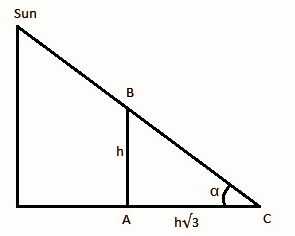In triangle CAB,

tan ɑ = BA / CA

tan ɑ = h / h√3

tan ɑ = 1 / √3

ɑ = 30o

The elevation angle of the sun is 30o.

Question 6: Write the locus of the points equidistant from the two given points.

Solution: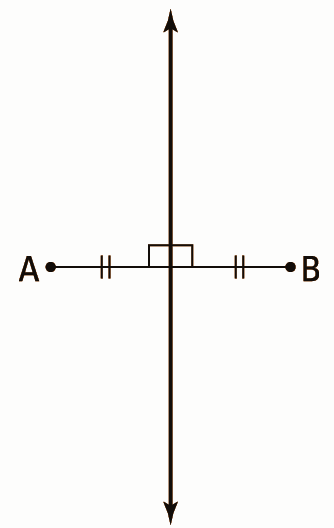The locus of points equidistant from two given points is the perpendicular bisector of the segment that joins the two points.

Question 7: Find the ratio between the chords which are equidistant from the centre of a circle.

Solution:

The chords are equidistant from the centre are equal.

Let the chords be AB, CD

Centre of the circle O.

Let the midpoints of chords be X, Y.

Now, OX = a, OY = b.

Also, AB = c, CD = d.

Given, OX = OY

So, a = b.

If a = b , AB = CD

That is c = d.

From the question,

AB / CD = c / d = c / c = d / d = 1

Therefore, the ratio of chords which are equidistant from the centre is 1.

Question 8: A dice is thrown once. Find the probability of getting an odd number.

Solution:

Total outcomes that can occur are 1, 2, 3, 4, 5, 6

Number of possible outcomes of a dice = 6

Numbers which are odd = 1, 3, 5

Total numbers which are odd = 3

Probability of getting an odd number

= (Number of outcomes where there is an odd) ÷ (Total number of outcomes)

= 3 / 6

= 1 / 2​

Question 9: In a city, the fare of a taxi for the first kilometre is Rs. 5 and after that, it is Rs. 3. If the distance covered is x km and fare is Rs. y, then express it in the form of the equation.

Solution:

Total distance covered is x.

Fare for 1st kilometer = Rs. 5

Fare for the rest of the distance = Rs. (x – 1) 3

Total fare = Rs. 5 + [x – 1]3

y = 5 + 3x – 3

y = 3x + 2

3x – y + 2 = 0

Hence, the value of the equation is 3x – y + 2 = 0.

Question 10: If the elevation angle of a camera situated at the top of a pole from a point 20 metre away from the base of the pole is 60o, find the height of the pole.

Solution: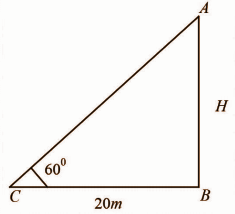tan 60o = AB / BC

√3 = H / 20

H = 20√3 m

PART – B

Question 11: Find the square root of 6889 by using Dwandwa Yoga Method.

Solution:

Simplify the expression,

Using the Dwandwa yoga method,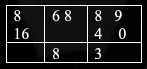Step 1: First square root 8 and remainder = 68 – 64 = 4.

8 * 2 = 16

Step 2: New dividend 48 ÷ 16 = 3, remainder = 0.

Implement dividend 9 – 32 = 0.

Hence, the value is 83.

Question 12: If the product of two numbers is 525 and their H.C.F. is 5, then find their L.C.M.

Solution:

Simplify the expression,

HCF (A, B)* LCM(A, B) = Product of A and B.

5 * LCM = 525

LCM = 525 / 5

LCM = 105

Question 13: The total surface area of a cube is 216 square metre. Find the side of the cube.

Solution:

The total surface area of the cube is 216 cm2.

The total surface area of the cube is 6a².

6a² = 216

=> a² = 216 / 6

=> a² = 36

=> a = 6

Therefore, the side of the given cube is 6cm.

Question 14: The radius of a semi-sphere is 7 cm, find the total surface area of it.

Solution:

The total surface area of a semi-sphere is 3𝛑r2.

TSA = 3 * (22 / 7) * (72)

= 3 * 22 * 7

= 462 cm2

Hence, the total surface area of the semi-sphere is 462cm2.

Question 15: A CCTV camera is placed on the top of a 24 m high pole in such a way that traffic can be seen beyond 25 metres of the line of sight of it. Find the area of the Green patch around the pole.

Solution: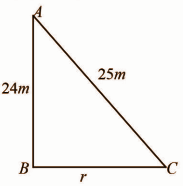Using Pythagoras theorem,

252 = 242 + r2

r2 = 252 – 242

= 625 – 576

= 49

r = 7m

Area of the green patch around the pole = 𝛑r2

= (22 / 7) * (72)

= 22 * 7

= 154m2

Question 16: By using the division algorithm method find quotient and remainder when polynomial P(x) = x4 – 3x2 + 4x – 3 is divided by g(x) = x2 + 1 – x.

Solution: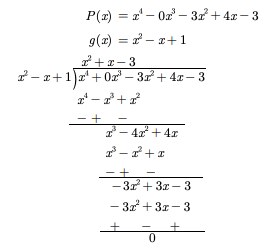p(x) = g(x) * q(x) + r(x)

x4 – 3x2 + 4x – 3 = [x2 + 1 – x] [x2 + x – 3] + 0

= x4 – 3x2 + 4x – 3

Hence, x4 – 3x2 + 4x – 3 and remainder 0.

Question 17: If the second and third terms of an Arithmetic Progression are 3 and 5 respectively, then find the sum of the first 20 terms of it.

Solution:

Simplify the A.P expression,

Second and third terms of an Arithmetic Progression are 3 and 5.

a = 3

d = 5 – 3 = 2

n = 20

d = a2 – a1

2 = 3 – a1

2 – 3 = -a1

-1 = -a1

a1 = 1

Sn = [n / 2] (2a + [n – 1]d)

= [20 / 2] [2 * 1 + [20 – 1] * 2]

= 10 [2 + 38]

= 400

S20 = 400

Question 18: The angles of elevation of the top of a tower from two points at a distance of 9 m and 25 m from the base of the tower in the same straight line are complementary. Find the height of the tower.

Solution: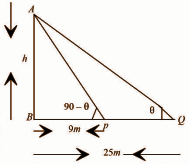In the triangle ABP,

h / 9 = tan θ —- (1)

h / 9 = tan (90 – θ) = cot θ

In triangle ABQ,

h / 25 = tan θ —- (2)

Multiply equation (1) and (2),

[h / 9] [h / 25] = cot θ * tan θ

h2 = 9 * 25

h2 = 225

h = 15m

The height of the tower is h = 15m.

Question 19: In the given figure if OP * OQ = OR * OS, then show that ∠OPS = ∠ORQ and ∠OQR = ∠OSP.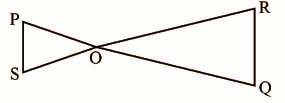Solution:

In the triangle POS and ROQ,

OP / OS = OR / OQ

∠POS = ROQ [vertically opposite angles]

So, POS and ROQ are congruent triangles by SAS and their corresponding angles are equal.

Therefore, ∠OPS = ∠ORQ and ∠OQR = ∠OSP.

Question 20: In a triangle ABC, the medians AD, BE and CF pass-through point G. If AD = 9 cm, GE = 4.2 cm and GC = 6 cm, then find the values of the lengths of AG, BE and FG.

Solution: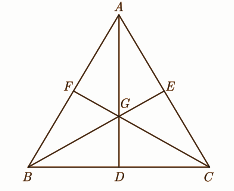AG / GD = 2 / 1

GD / AG = 1 / 2

[GD / AG] + 1 = [1 + 2] / 2

9 / AG = 3 / 2

AG = [9 * 2] / 3 = 6cm

BG / GE = 2 / 1

[BG / GE] + 1 = [2 / 1] + 1

[BG + GE] / GE = [2 + 1] / 1

BE / GE = 3 / 1

BE = 3 * GE = 3 * 4.2 = 12.6cm

FG / GC = 1 / 2

FG = [1 / 2] GC

= [1 / 2] * 6

= 3cm

Question 21: In the given figure some angles are represented by x, y and z. Find the values of these angles.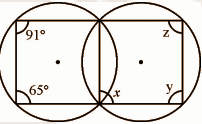Solution: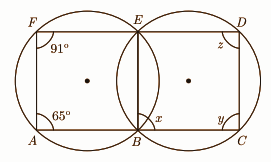65 + x = 180o

x = 180 – 65

x = 115o

Since, ∠AFE = ∠CBE

91o = x

x + z = 180o

z = 180 – x

= 180 – 91

z = 89o

Question 22: Draw two tangents PA and PB from an external point P to a circle of radius 4 cm, where the angle between PA and PB is 65o.

Solution: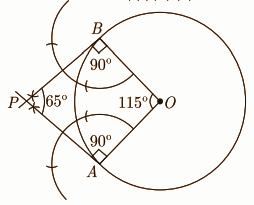Question 23: The radius of a circular park is 4.2 m. A path of 1.4 m width is made around the circular park. Find the area of the path.

Solution:

Radius (R) of circular park = 4.2 m

Area of the circular park = πr²

= [22 / 7] * 4.2 * 4.2

= 55.44 m²

Width (r) of the circular path = 1.4 m

Area of the circular park including the circular path = π(R + r)² sq.m

= [22 / 7] * (4.2 + 1.4)²

= [22 / 7] * 5.6 * 5.6

= 98.56 m²

Area of Path = Area of the park incl. path – Area of the path

= 98.56 – 55.44

= 43.12 m²

Question 24: The length and diameter of a roller are 2.5 m and 1.4 m, respectively. How much area will be planned by roller in 10 revolutions?

Solution:

Diameter of the roller = 1.4 m

Radius of the roller = 0.7m

Length of the roller = 2.5 m

Area covered by the roller in 1 revolution = Curved surface area of the roller

= 2πrh

= 2 × [22 / 7] × 0.7 × 2.5

= 11 m2

Area covered by the roller in 10 revolutions = 11 × 10 = 110 m2.

Question 25: In a bag one white ball, two black balls and three red balls of the same size are placed.

A ball is drawn at random from this bag. Find the probability:

(i) the ball is white

(ii) the ball is not black

(iii) the ball is red

Solution:

Total number of all possible outcomes = 1 + 2 + 3 = 6

(i) Number of white ball = 1

P (getting a white ball) = 1 / 6

(ii) Number of balls that are not black = 1 + 3 = 4

P (not drawing a black ball) = 4 / 6 = 2 / 3

(iii) Number of red balls = 3

P (drawing a red ball) = 3 / 6 = 1 / 2

Question 26: Solve the following pair of linear equations by graphical method:

2x + y = 6, 2x – y = 2. Thus find the value of p in the relation 6x + 7y = p.

Solution:

2x + y = 6 —- (1)

2x – y = 2 —- (2)

4x = 8

x = 2

Put x = 1 in (1),

2 * 2 + y = 6

y = 6 – 4

y = 2

In 6x + 7y = p

6 * 2 + 7 * 2

= 12 + 14

= 26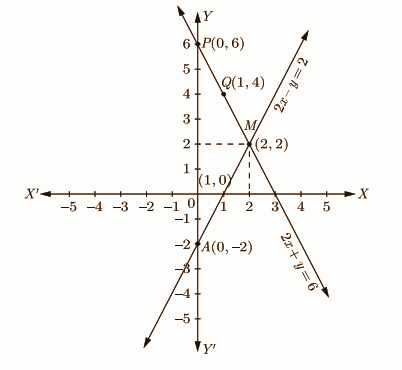Question 27: Prove that:

[i] √[1 + cos θ] / [1 – cos θ] = cosec θ + cot θ

[ii] tan θ / [1 – cot θ] + cot θ / [1 – tan θ] = 1 + tan θ + cot θ

OR

[i] If sin θ + cos θ = p and sec θ + cosec θ = q, then prove that q(p2 – 1) = 2p.

[ii] Prove that:

cos A / [1 – tan A] + sin A / [1 – cot A] = sin A + cos A

Solution:

[i] √[1 + cos θ] / [1 – cos θ]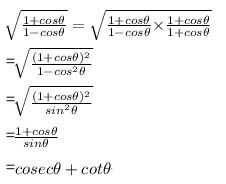[ii] tan θ / [1 – cot θ] + cot θ / [1 – tan θ] = 1 + tan θ + cot θ

LHS = tanθ / (1 – cotθ) + cotθ / (1 – tanθ)

= tanθ / (1 – 1 / tanθ) + (1 / tanθ) / (1 – tanθ)

= tan²θ / (tanθ – 1) + 1 / tanθ (1 – tanθ)

= tan³θ / (tanθ – 1) – 1 / tanθ (tanθ – 1)

= (tan³θ – 1) / tanθ (tanθ – 1)

= (tanθ – 1)(tan²θ + 1 + tanθ) / tanθ (tanθ – 1)

= (tan²θ + 1 + tanθ) / tanθ

= tanθ + cotθ + 1

OR

[i] If sin θ + cos θ = p and sec θ + cosec θ = q, then prove that q(p2 – 1) = 2p.

Consider, sec θ + cosec θ = q

⇒ [(1 / sinθ) + (1 / cosθ)] = q

⇒ [(sinθ + cosθ) / sinθ cosθ] = q

⇒ [p / sinθ cosθ] = q

⇒ sinθ cosθ = p / q → (1)

Consider, sinθ + cosθ = p

Squaring on both the sides we get

(sinθ + cosθ)2 = p2

⇒ sin2θ + cos2θ + 2 sinθ cosθ = p2

⇒ 1 + 2 (p / q) = p2 [From (1)]

⇒ (q + 2p) / q = p2

⇒ (q + 2p) = p2q

⇒ 2p = p2q – q

⇒ 2p = q(p2 – 1)

[ii] cos A / [1 – tan A] + sin A / [1 – cot A] = sin A + cos A

cos A / (1 – tan A) + sin A /(1 – cot A) = sin A + cos A

LHS = cos A / (1 – tan A) + sin A /(1 – cot A)

= cos A / (cos A – sin A) + sin A / (sin A – cos A)

= cos A / (cos A – sin A) – sin A / (cos A – sin A)

= (cos A – sin A) / (cos A – sin A)

= (cos A + sin A) (cos A – sin A) / (cos A – sin A)

= (cos A + sin A)

Question 28:

[i] If the distance between points (x, 3) and (5, 7) is 5, then find the value of x.

[ii] Find the ratio in which the line 3x + y = 9 divides the line segment joining the points (1, 3) and (2, 7).

Solution:

[i] Let (x1, y1) = (x, 3)

(x2, y2) = (5, 7)

d = √(x2 – x1)2 + (y2 – y1)2

5 = √(5 – x)2 + (7 – 3)2

d = 5

On squaring of both sides,

25 = (5 – x)2 + 16

25 – 16 = (5 – x)2

9 = (5 – x)2

(5 – x) = ± 3

If x = 3,

5 – x = 3

5 – 3 = x

x = 2

If x = -3,

5 – (-3) = 3

x = 8

x = 2, 8

[ii] Let the line divides the points in k:1 ratio according to section formula

(2k + 1 / k + 1, 7k + 3 / k + 1) = (x, y)

It must satisfy the given equation so

3(2k + 1 / k + 1) + (7k + 3 / k + 1) = 9

6k + 3 + 7k + 3 / k + 1 = 9

13k + 6 = 9k + 9

13k – 9k = 9 – 6

4k = 3

k = 3 / 4

Hence, the ratio is 3:4.

Question 29: [i] ABC is a right-angled triangle whose angle B is a right angle. If points D and E are situated on the sides AB and BC, respectively, then prove that AE2 + CD2 = AC2 + DE2.

OR

[ii] If two sides of a cyclic quadrilateral are parallel, then prove that other sides are equal and its diagonals are also equal to each other.

Solution:

[i]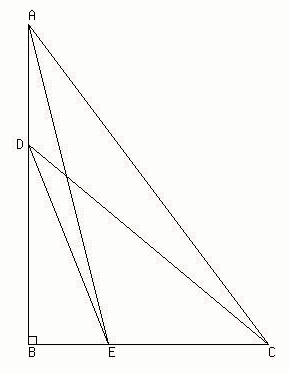ΔABE is a right triangle, right-angled at B

AB² + BE² = AE²……………..(1) (by the Pythagoras theorem)

ΔDBC is a right triangle, right-angled at B

DB² + BC² = CD²…………….(2) (by the Pythagoras theorem)

AE² + CD² = (AB² + BE²) + (BD² + BC²)

AE² + CD² = (AB² + BC²) + (BE² + BD²)……(3) [Rearranging the terms]

ΔABC is a right triangle,

AB² + BC² = AC²…………….(4) (by the Pythagoras theorem)

ΔDBE is a right triangle

DB² + BE² = DE²………………(5) (by the Pythagoras theorem)

AE² + CD² = (AB² + BC²) + (BE² + BD²)

AE² + CD² = AC² + DE²

OR

[ii]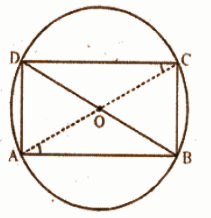AB || DC ⇒ ∠DCA = ∠CAB [alternate angles]

Now, chord AD subtends ∠DCA and chord BC subtends ∠CAB at the circumference of the circle.

∠DCA = ∠CAB [proved]

Now in triangle ABC and ADB,

AB = AB [common]

∠ACB = ∠ADB [angles in the same segment]

By SAS criterion of congruence,

The corresponding parts of the congruent triangles are congruent.

AC = BD

Question 30: Find the mean and mode of the following frequency distribution: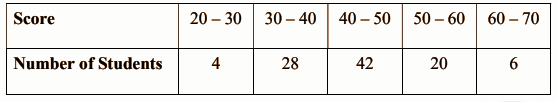Solution:

 Score Number of students Mid value (fi * xi) 20 – 30 4 25 100 30 – 40 28 35 980 40 – 50 42 45 1890 50 – 60 20 55 1100 60 – 70 6 65 390 N = 100 4460

Mean = 4460 / 100

= 44.6

Hence, the mean is 44.6.

As the lifetime (in hours) 40 – 50 has a maximum frequency, so it is the modal lifetime in hours.

xk = 40, h = 10, fk = 42, fk-1 = 28, fk+1 = 20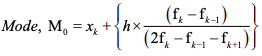= 40 + [10 * [42 – 28] / [2 * 42 – 28 – 20]]

= 40 + [3.89]

= 43.89

Hence, the mode is 43.89.